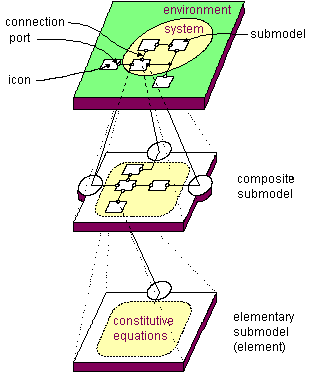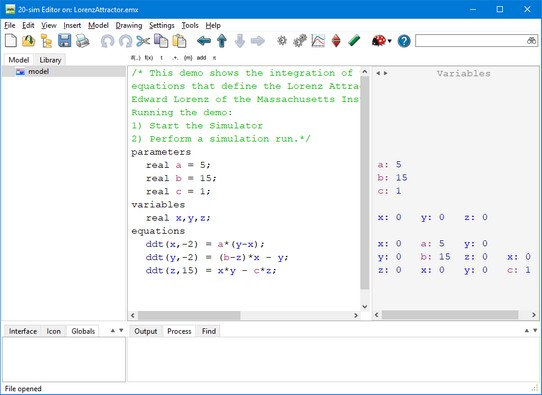﻿ 20-sim webhelp > Language Reference > Introduction > Language Reference

# Language Reference

Every (sub)model has an implementation. This can be a composition of lower level submodels, which themselves are composed of lower level submodels etc. At the bottom of this hierarchy the submodels consist of a set of mathematical equations (equation submodel). These submodels are therefore known as equation submodels.All equations used in 20-sim are described in the language SIDOPS+. A simple equation model written in SIDOPS+ is shown below:A simple equation model in 20-sim.

You will find out that in most cases the SIDOPS+ language is equal to standard mathematical notation. Regardless of your modeling background, you can learn quickly to build your own equation models. 20-sim comes with over 80 built-in functions that do most of the work for you. To learn more about equation models, have a look at the following sections:

 1 Model Layout
 2
 3 Data Types
 4 Operators
 5 Functions
 6 Statements